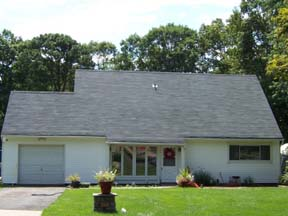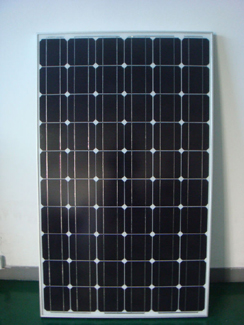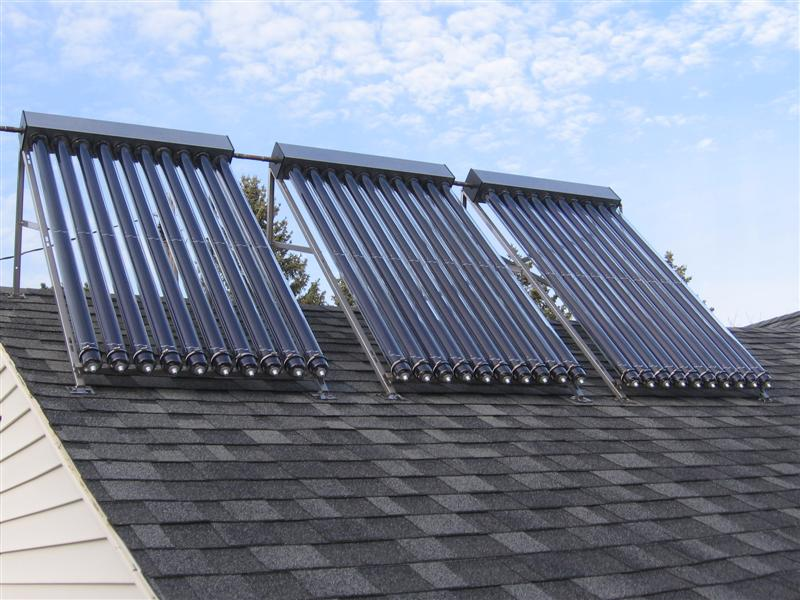HOME Hobby Corner Dave's Circuits Electronic Resources Book Corner Contact Info

 Back of the Envelope Previous Issues

Solar Energy Myth Busting I

I’m sure you have heard this myth.  “The average home roof receives enough solar energy each day to provide all the electrical, heat and air-conditioning needs inside the home.”

I always thought that such a statement was factual.  But is it really true?  Let’s do some quick math and find out.An ideal roof faces due south and is slanted at about 45 degrees.  A ranch style home might have a footprint of about 3000 square feet.  But, only half the roof area would be facing south.  So, we will calculate the possible solar energy collection with 1500 square feet of exposed roof area.  Typical sunlight has about 100 watts of power per square foot of area and the typical sunlit day will last 6 hours.  So, the total energy hitting our hypothetical roof is 1500 x 100 x 6 or 900KW-hours.  OK, so that is the amount of solar energy on the roof for a day.   Now, how much energy does the home need?

I figure the typical home needs about 25 kilowatt-hours of electricity each day. In cold climates, the natural gas needed for heating the home and heating domestic water per day might be about 1M BTUs.This estimate is based on my own usage, which might be a bit more than average. One BTU is equal to 0.0003 kilowatt-hours.  So, 1M X 0.0003 equals 300KW-hours.  Adding both the electrical and heating needs, we come up with a rough figure of 325KW hours of energy needed per day for our house.  This is about 30% of the total energy hitting the roof.  So, the myth is confirmed.  In fact, our hypothetical house would receive three times more solar energy on its roof than needed inside.

Now, what is the practical amount of energy which could be collected from all that sunlight?
To crank out 25KW-hours of energy in 6 hours, the solar panels would have to generate 25KW divided by 6 or 4KW of power.  Some of the best solar panels available today have an efficiency greater than 15%.  So, for each square foot of area the panels would produce 15 watts of electrical power.  To generate 4KW, the exposed solar panel area would have to be at least 4000 watts divided by 15 or 270 square feet of panel area. A typical 250 watt panel would be about 3 feet by 5 feet.4000 divided by 250 is 16 panels at a minimum but Let’s toss in two more panels to cover overall losses.    That means the 18 panels and would take up 270 square feet of roof area.  That leaves 1200 more square feet of the 1500 square feet of the total roof area left for heat collection.250 Watt Solar Panel Roof Mounted Heat Collectors

Using sunlight to heat water is one of the most efficient ways to tap into this energy source.  Even in cold climates I figure that a collector should be able to pull in some 250 watt-hours per square foot per day.  That would put the efficiency at about 40%. Many systems claim even a higher efficiency.0.25KW-hours X 1200 square feet yields 300KW-hours for the total roof area per day.  If we add the 25KW-hours from the solar panel, we get a total of 325KW-hours.  Reviewing the above calculations, this figure is exactly the total amount of energy needed by the home.  So it sure looks like it would be possible to for a well designed solar energy system to keep a house running without tapping into any additional power source.  But, the sun does not shine all day.  To provide a continuous energy flow into the house at night, excess electrical energy made during the day would flow into a storage battery and excess heat energy would be stored in large insulated tanks of water.  Real “off-grid” homes will also include a gasoline power back-up generator and may also have one or more wind generators for additional power sources.

In conclusion, yes it is possible and practical to collect enough solar energy from the roof of a typical home to power everything inside.  As this technology improves, we should see the overall efficiency of both photovoltaic and solar heat collectors increase. There are already some hybrid systems which combine photovoltaic panels with heat collectors to further increase the overall efficiency.  The cost of these systems is also rapidly coming down.

 January 2011 Page 1 Back Envelope Circuit Diagnosis Experimenter's Corner Good Idea done Badly What the World needs Now Wily Widget